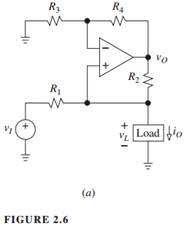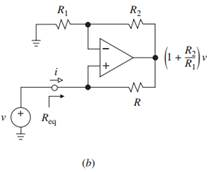### Create an Account

Home / Questions / If the Howland current pump of Fig 2 6a is implemented with four 10-kΩ resistances and an...

# If the Howland current pump of Fig 2 6a is implemented with four 10-kΩ resistances and an op amp having a0 105 V/V ft 1 MHz rd = and ro = 0 sketch and label the magnitude plot of the

If the Howland current pump of Fig. 2.6a is implemented with four 10-kΩ resistances and an op amp having a= 105 V/V, ft = 1 MHz, r= ∞, and r= 0, sketch and label the magnitude plot of the impedance Zo seen by the load. Justify using physical insight.2. The negative-resistance converter of Fig. 1.21b is implemented with three 10-kΩ resistances and an op amp with GBP = 1 MHz. Find its impedance Zeq. How does it change as f is swept from 0 to ∞?Aug 06 2020 View more View LessSubscribe To Get Solution